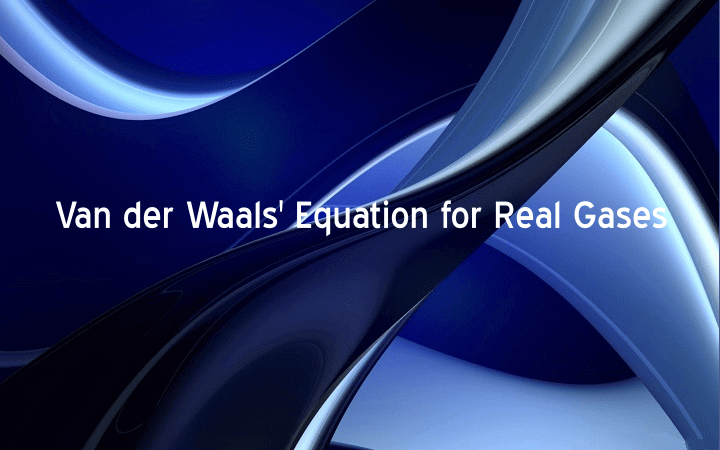## Van der Waals’ Equation for Real Gases

The general gas equation is not applicable to real gases at the all the temperature .and pressure. In order to make it applicable to real gases Van der Waals’ made correction in volume and pressure of the gases and derived an equation known as Van […]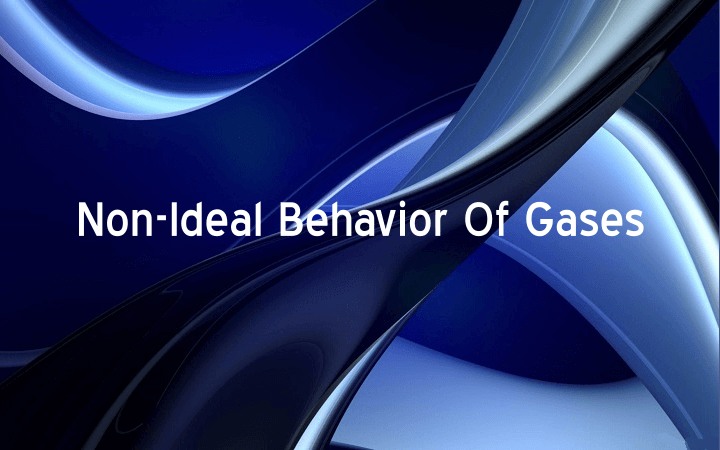## Non-Ideal Behavior Of Gases

Ideal Gases These are the gases, which obey gas laws at all temperatures and pressures. No such gas is known. Non-Ideal gases (Real gases) These are the gases, which obey gas laws but only at high temperatures and low pressures. These gases do not obey […]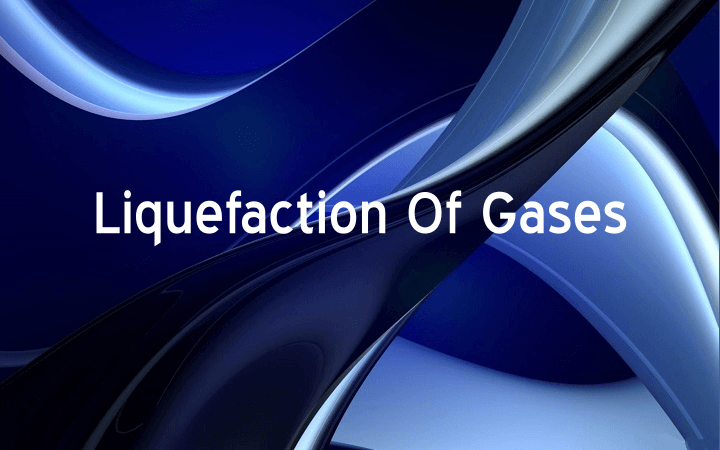## Liquefaction Of Gases

General Principle of Liquefaction The liquefaction of a gas requires high pressure and low temperature. The pressure brings the molecule of a gas close to each other. Low temperature causes to increases attractive forces. For every gas there is a temperature above which the gas […]## Kinetic Equation

Definition: R.J. Clausius deduced an equation for the pressure of an ideal gas from the above assumptions of kinetic theory. This equation is called kinetic equation. Mathematical equation: Explanation: Since the molecules of a gas under the given conditions don’t have the same velocity, therefore […]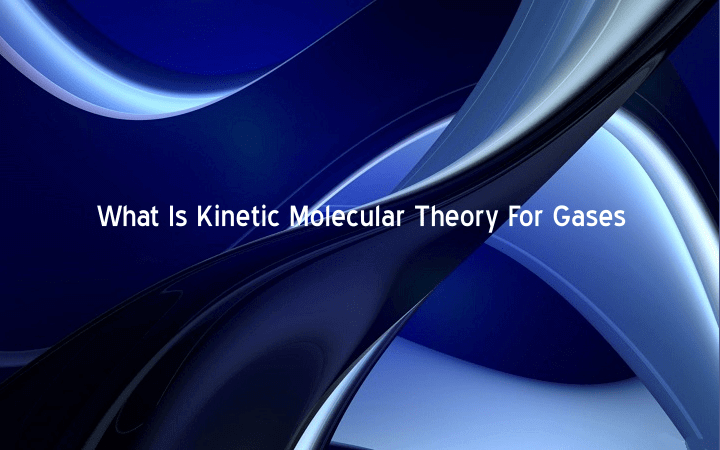## What Is Kinetic Molecular Theory For Gases

Behavior of all the gases is similar to each other. To explain the similar behavior of all the gases, a model was devised. This model is known as kinetic molecular theory of gases. Main Postulates: The postulates of this theory are: Gases are made up […]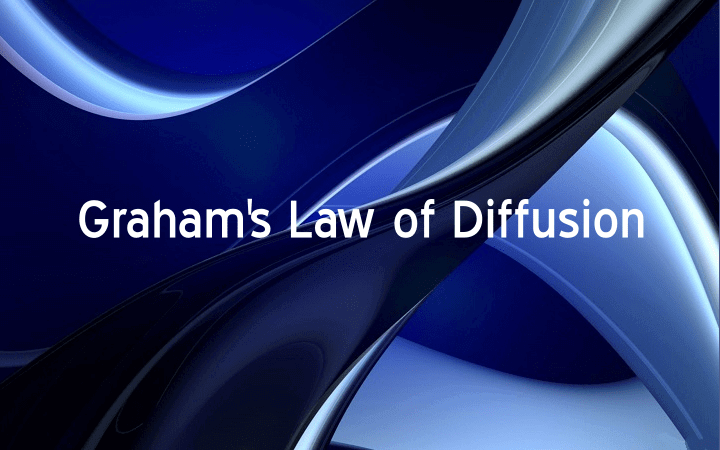## Graham’s Law of Diffusion Definition

“The rates of diffusion of two gases are inversely proportional to the square roots of their densities or molecular weights at the same temperature and pressure”. Mathematical formula: Graham’s Law of Diffusion Equation, Where r1 and r2 are the rates of diffusion of two gases, […]